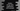# Python string index method explanation with example## Python string index method:

index is an inbuilt method in the python string class. This method is used to find the first occurrence of a value in a given string. In this post, we will learn how to use index method with example.

### Definition of index method:

index method is defined as below:

``str.index(s, start, end)``

Here,

• s is the string to find in the string str
• start is the start index to start the search. It is an optional value. By default, it takes 0 or it starts the search from the beginning.
• end is the end index of the search. By default it searches to the end of the string and it is an optional value.

This method throws one ValueError if the provided string is not found.

``ValueError: substring not found``

Let me show you how it works with examples.

### Example 1: Without using start and end index:

Let’s take a look at the below program:

``````given_text = 'hello World !!'

print(given_text.index('World'))
print(given_text.index('o'))``````
• The first print statement prints the first index of the word World in the string given_text.
• The second print statement prints the first index of the character o in given_text.

It will print:

``````6
4``````

### Example 2: With start and end index:

Let’s take a look at the example below:

``````given_text = 'hello World !!'

print(given_text.index('l'))
print(given_text.index('l', 3))
print(given_text.index('l', 7, 12))``````

Here,

• all print statements are printing different values.
• The first print statement finds the index of the first l in the string given_text
• The second print statement finds the index of the first l in the string given_text from start index 3 to the end of the string.
• The first print statement finds the index of the first l in the string given_text from start index 7 to index 12.

It will print the below output:

``````2
3
9``````